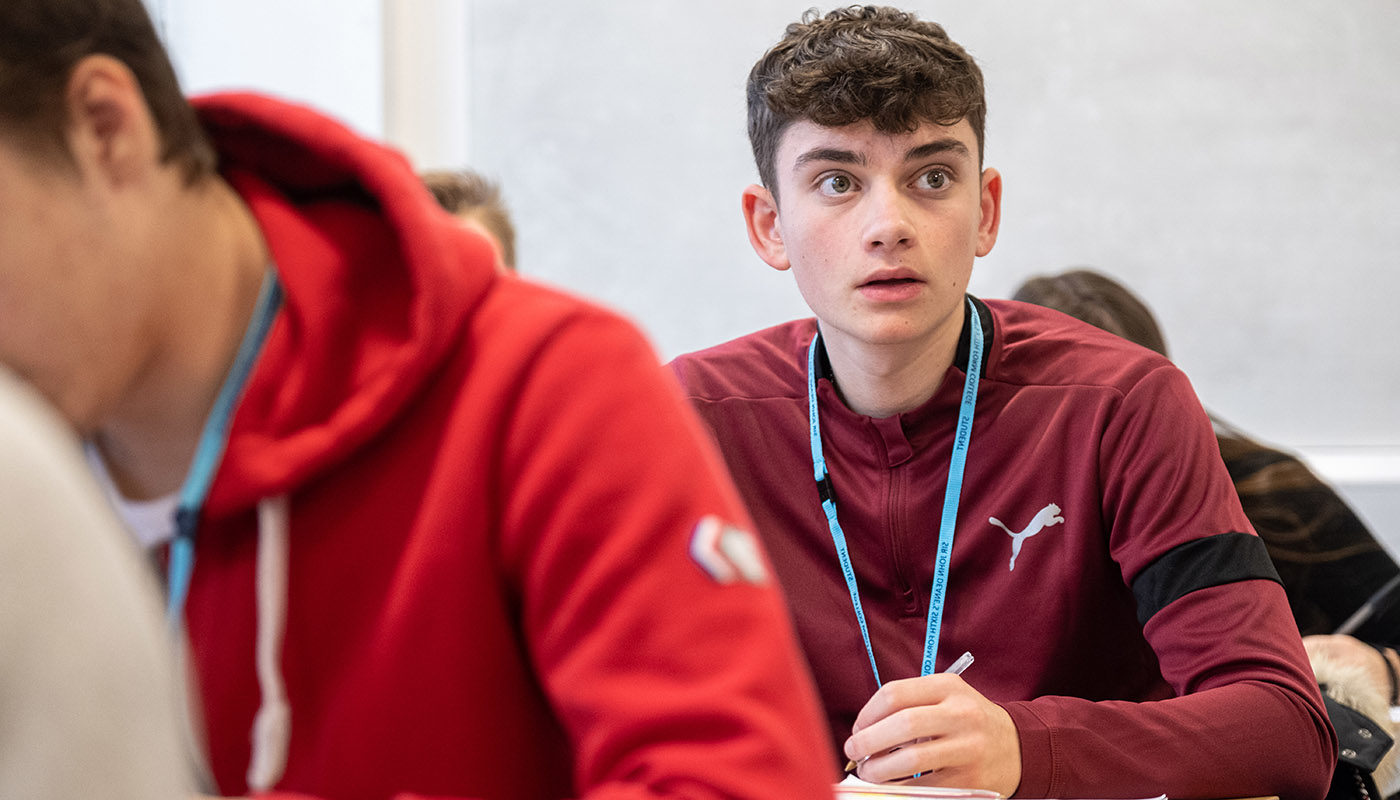# Further Mathematics

## Further Mathematics is an enjoyable, rewarding and stimulating qualification, which both extends and deepens your knowledge and understanding beyond the standard A level Mathematics.Further Mathematics introduces new topics such as matrices and complex numbers that are vital for studying STEM subjects at university.

Half of the course looks at pure mathematics, while the other half is made up of mechanics and statistics. We follow the Edexcel specification for this course. Students will sit four papers at the end of their second year, each lasting for one hour 30 minutes. There is also an opportunity to complete an AS in Further Maths. Students completing this option will be examined at the end of their first year.

As part of our commitment to ensuring students enjoy the challenges that studying Mathematics brings, we arrange a number of activities throughout the year to enrich their experience.

These include attending problem-solving days at local universities, entering a range of individual and team challenges both locally and nationally and attending residential sessions at local universities with a view to undergraduate study of Mathematics. Students can also enter and be supported with preparing for a number of examinations that are required for university entry, such as STEP, MAT, BMAT or AEA.

Studying Further Mathematics is challenging but extremely rewarding and stimulating and provides a great foundation for going on to study for a degree in a STEM subject.

If you are planning to take a degree with a strong mathematical content, such as Mathematics, Engineering, Sciences, Computer Science or Finance/Economics, you will benefit enormously from taking Further Mathematics.

Many students go on to enjoy careers in mathematics, engineering, physics, computer science or financial services.

Pure Mathematics- Exam Paper – 25% of marks

Pure Mathematics – Exam Paper – 25% of marks

Statistics – Exam Paper – 25% of marks

Mechanics – Exam Paper – 25% of marks

Summary

Pure Mathematics
Proof; Complex Numbers; Matrices; Algebra and Functions; Further Calculus; Vectors; Polar Coordinates; Hyperbolic Functions; Differential Equations.

Statistics
Discrete Probability Distributions; Poisson and Binomial Distributions; Geometric and Negative Binomial Distribution; Hypothesis Testing; Central Limit Theorem; Chi Squared Test; Probability Generating Function; Quality of Tests.

Mechanics
Momentum and Impulse; Work, Energy and Power; Elastic Strings, Springs and Elastic Energy; Elastic Collisions in one Dimension; Elastic Collisions in two Dimensions.

Students should be confident in applying Higher Level GCSE techniques, in particular:
• Manipulating surds and indices.
• Solving linear equations.
• Solving quadratic equations: by factorising, using the formula and completing the square.
• Solving simultaneous equations by elimination and substitution. Students should review these topics and practise questions on them using GCSE revision guides/the internet.

Students will also need to purchase a CASIO fx-991 EX (ClassWiz) scientific calculator. Other calculators are unfortunately not suitable. This is a new calculator designed for the new specification and includes statistical functions that are not present on other scientific calculators.

Study Level

A Level

Exam Board

Edexcel

Contact Details

Mr M Griffiths